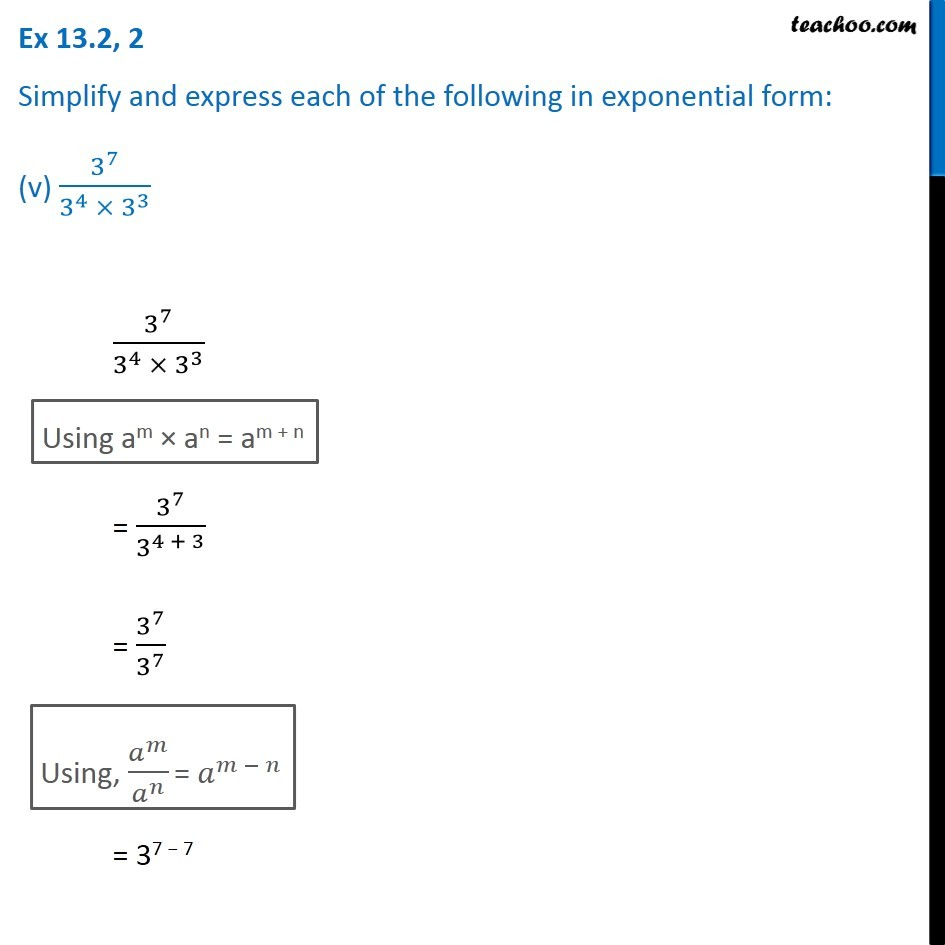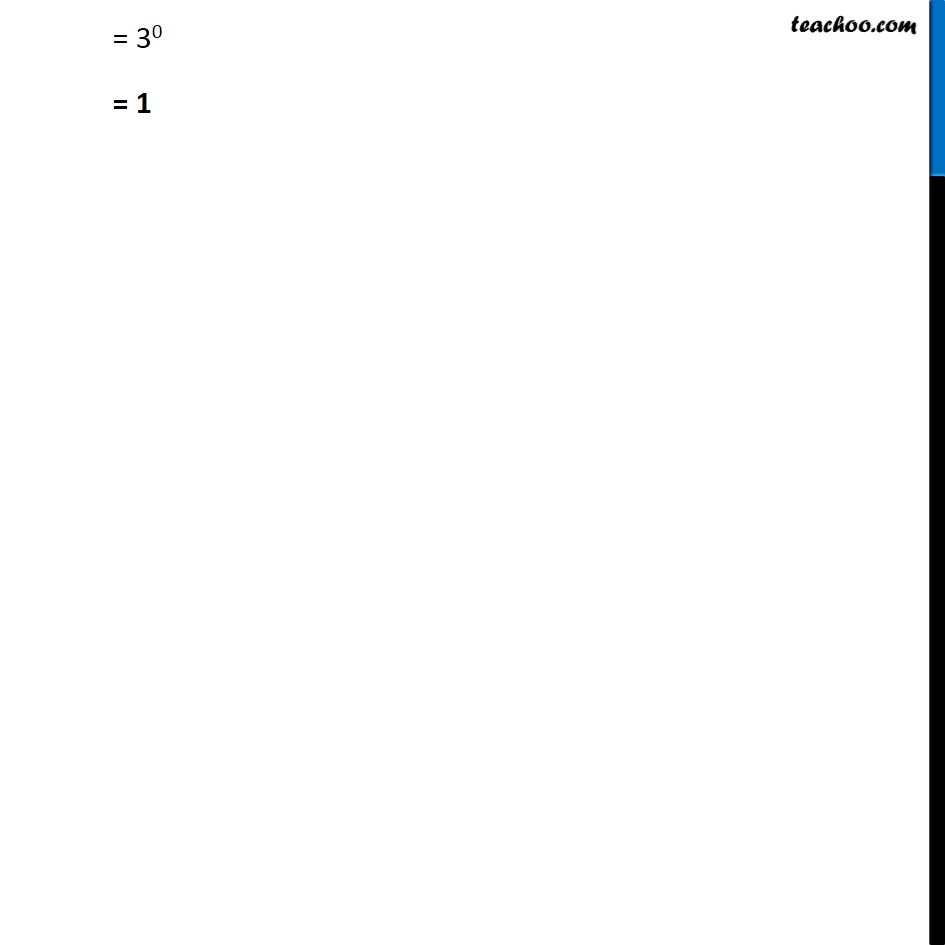1. Chapter 13 Class 7 Exponents and Powers
2. Serial order wise
3. Ex 13.2

Transcript

Ex 13.2, 2 Simplify and express each of the following in exponential form: (v) 3^7/(3^4 ×〖 3〗^3 ) 3^7/(3^4 ×〖 3〗^3 ) Using am × an = am + n = 3^7/3^(4 + 3) = 3^7/3^7 Using, 𝑎^𝑚/𝑎^𝑛 = 𝑎^(𝑚 − 𝑛) = 37 − 7 = 30 = 1